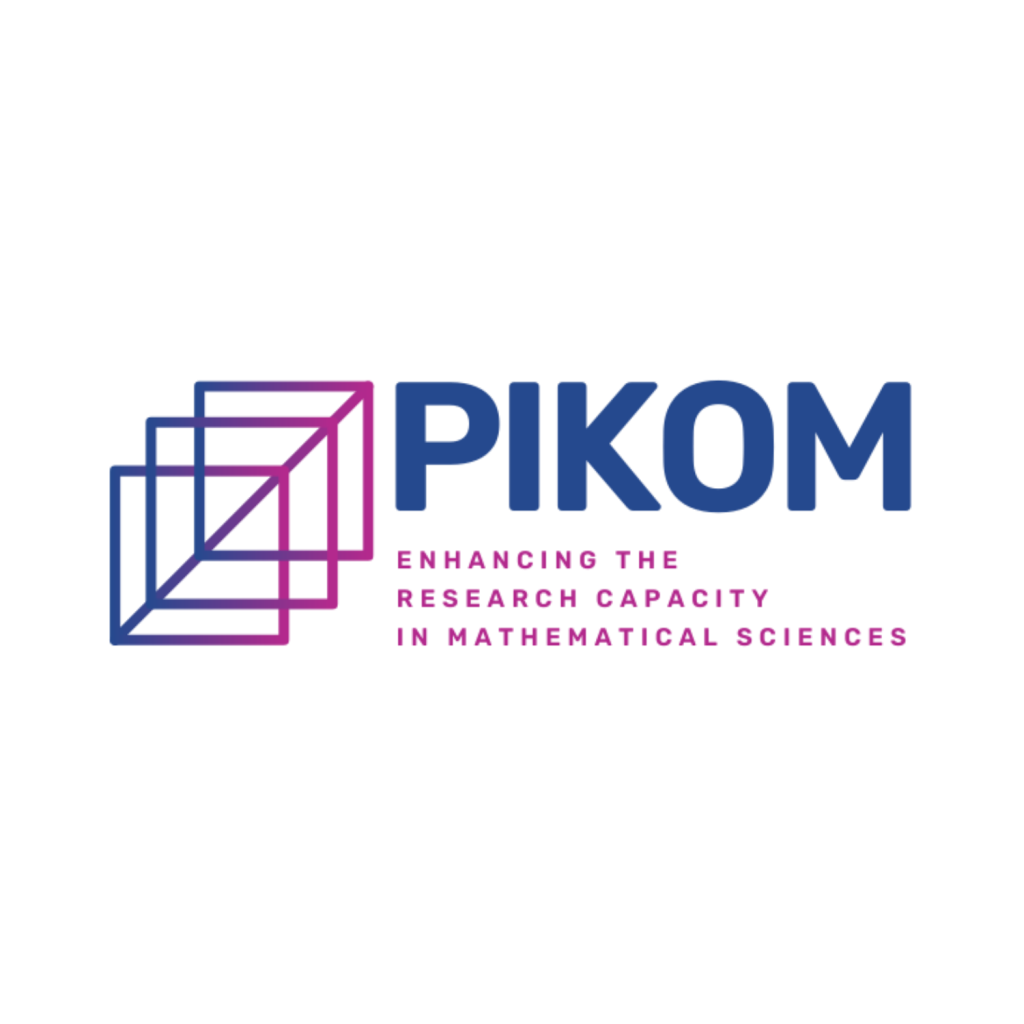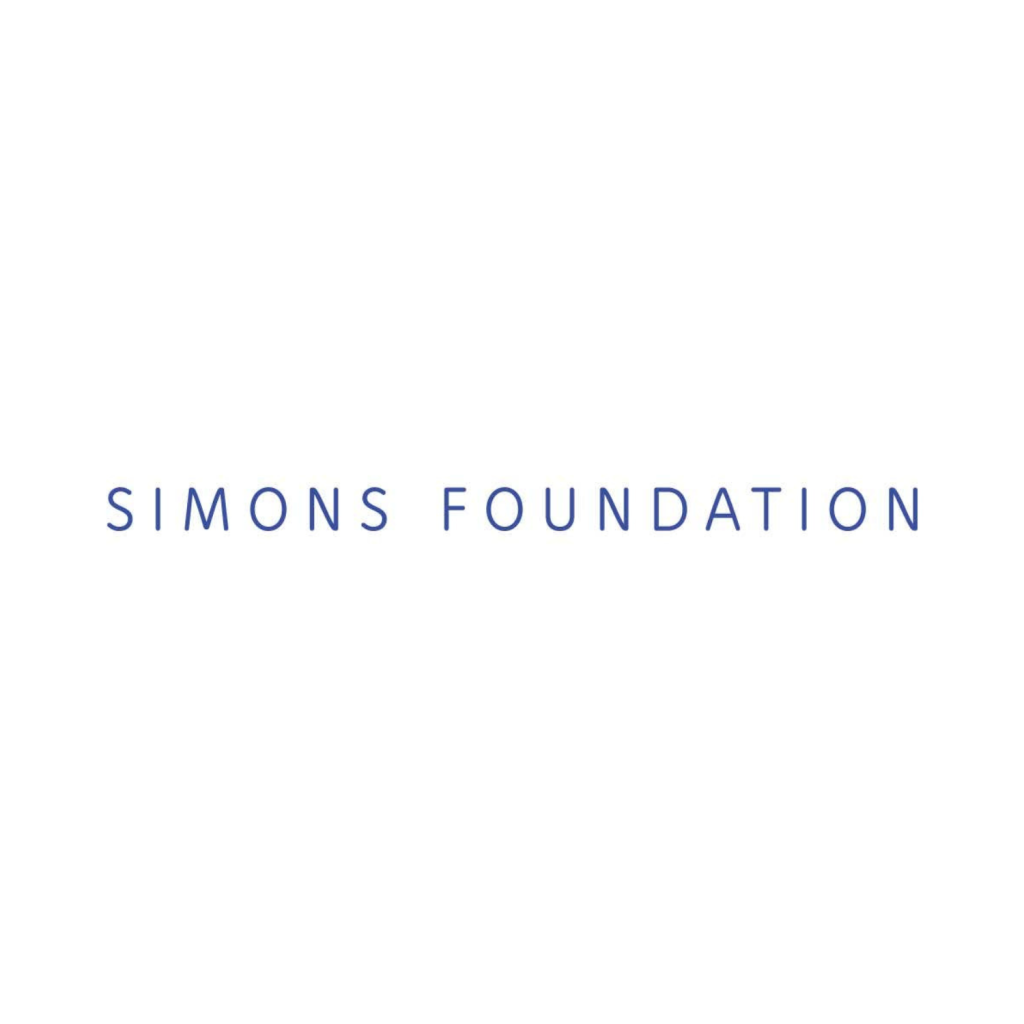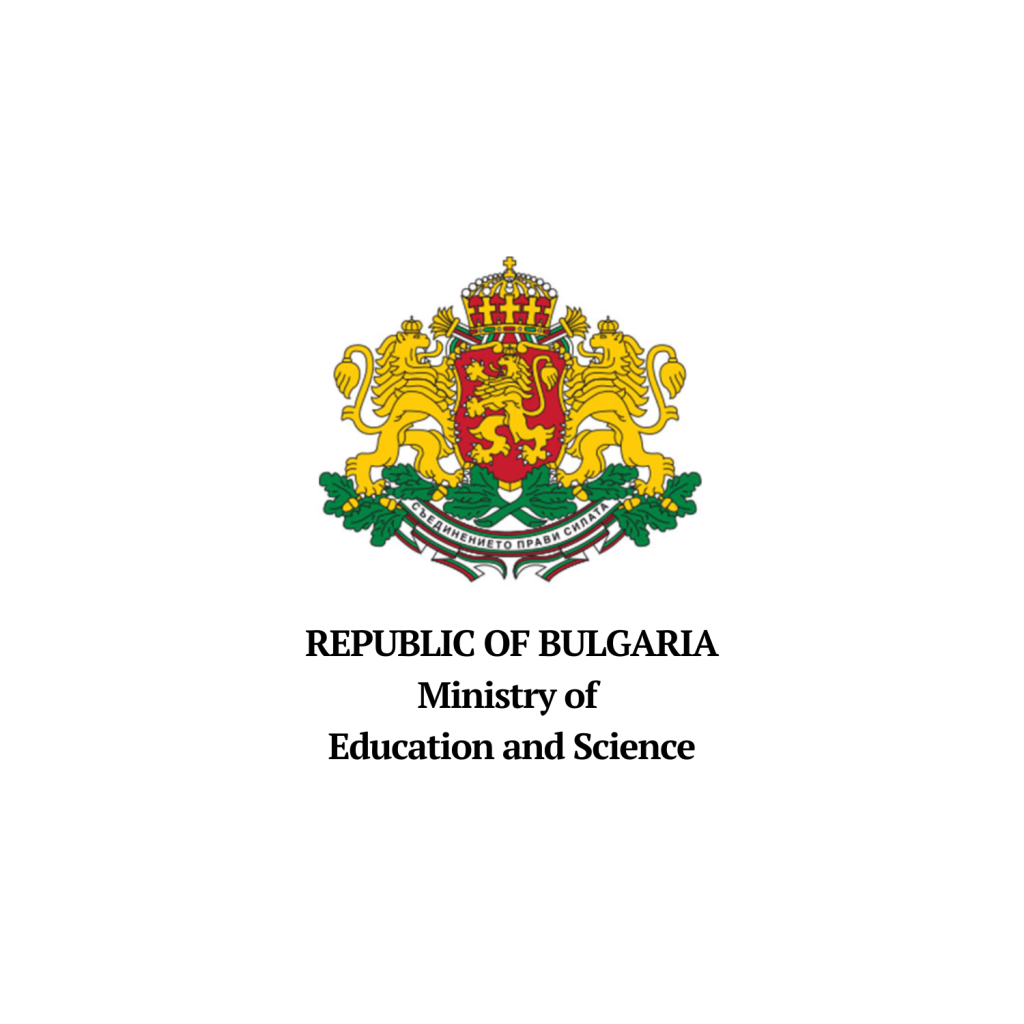# Section “Geometry and Topology”

### Participants

#### Invited Speakers

• Arkady Leiderman, Ben-Gurion University of the Negev, Israel

• Georges Dloussky, Professeur émérite, Université d’Aix-Marseille, Institut de Mathématiques de Marseille, France

• Ina Petkova, Dartmouth College, USA

• Julien Keller, Université du Québec à Montréal, Canada

#### Contributors

• Andrei Lasnier, Institute of Mathematics and Informatics, Bulgaria

• Danila Cherkashin, Institute of Mathematics and Informatics, Bulgaria

• Jose Medel Torrero, Florida International University, USA

• Peter Dalakov, American University in Bulgaria, Bulgaria

• Peter Petrov, Institute of Mathematics and Informatics, Bulgaria

• Stefan Ivanov, Sofia University “St. Kliment Ohridski”, Bulgaria

• Vesko Valov, Nipissing University, Canada

• Viktoria Bencheva, Institute of Mathematics and Informatics, Bulgaria

### Program and Abstracts

##### Arkady Leiderman - Kolmogorov Superposition Theorem and its applications in Topological Algebra

Let [0, 1] denote the unit closed segment. The classical Kolmogorov Superposition Theorem states that any d-variable continuous function defined on the unit d-dimensional cube [0, 1]d can be represented by means of superpositions of one-variable continuous functions. First, we briefly survey analytical and topological aspects of this celebrated result which resolved the Hilbert’s 13th problem.

Our research concerns to the structure of several free objects of Topological Algebra: free topological group F(X), free abelian topological group A(X), free locally convex space L(X), and free topological vector space V (X).

We will show how Kolmogorov Superposition Theorem can be effectively applied for the positive solution of the following question (see , , ):

Problem 1. Let X be any finite-dimensional metrizable compact space. Is it possible to embed isomorphically Φ(X) into Φ[0, 1], where Φ is a free topological functor listed above (Φ is A, L, V )? We will discuss some relevant questions which are still open. Consider the space Cp(X) of continuous real-valued functions on a compact space X equipped with the topology of pointwise convergence.

Problem 2. Find a complete characterization of compact spaces X such that there exists a continuous linear (linear and open) map from Cp[0, 1] onto Cp(X).

References:

 A. Leiderman, S.A. Morris and V. Pestov, The free abelian topological group and the free locally convex space on the unit interval, J. London Math. Soc. 56 (1997), 529–538.

 M. Krupski, A. Leiderman and S.A. Morris, Embedding of the free abelian topological group A(X ⊕ X) into A(X), Mathematika, 65 (2019), 708–718.

 A. Leiderman, S.A. Morris, Embeddings of free topological vector spaces, Bull. Austr. Math. Soc. 101 (2020), 311–324.

##### Georges Dloussky - On classification of non Kählerian compact complex surfaces

We address the question of the classification of compact complex surfaces with Betti numbers b_1=1 and b_2>0. We give some properties of the known surfaces, in particular the existence of Global Spherical Shells (GSS). It appears that the proof of the existence of Locally Conformally Symplectic (LCS) structures is a breakthrough which gives a strategy to end the classification.

This is a joint work with Vestislav Apostolov.

##### Ina Petkova - A contact invariant from bordered Heegaard Floer homology

To a Heegaard diagram presentation for a (closed, oriented) 3-manifold, one can associate a chain complex, whose homology is an invariant of the 3-manifold, called Heegaard Floer homology. Given a contact structure on the 3-manifold, one can refine this construction to obtain a special class in this homology, which is an invariant of the contact manifold. We will begin by outlining this construction.

The original definition of Heegaard Floer homology involves counting holomorphic curves in a high-dimensional manifold, and as a result the invariant can be hard to compute. Bordered Floer homology is a generalization of Heegaard Floer homology to manifolds with boundary, which provides powerful gluing techniques for computing the Heegaard Floer homology of closed 3-manifolds.

Given a contact 3-manifold with convex boundary, we describe an invariant which takes values in the bordered Floer homology of the manifold. This invariant satisfies a nice gluing formula, and recovers the contact class in Heegaard Floer homology.

This is joint work with Alishahi, Földvári, Hendricks, Licata, and Vértesi

##### Julien Keller - Constant scalar curvature Kähler cone metrics and beyond

We will review some recent progress on constant scalar curvature Kähler metrics with conical singularities along a divisor. We will discuss the existence of such special metrics, and provide some results on the particular case of projective bundles over a curve. Eventually, we will explain the relationship between such metrics and uniform log-K stability obtained by Aoi-Hashimoto-Zheng. If time allows, we will explain possible generalizations and applications to the twisted case.

##### Rafael López - New perspectives on the notion of catenary
The catenary is the shape of a hanging chain suspended from its endpoints. In this talk, we will give some topics around the catenary curve, such as its extension to higher dimensions and the study of catenaries in other ambient spaces.  As a special interest, we focus on the axisymmetric singular minimal surfaces, which are models of domes and roofs. We will discuss if catenary rotation surfaces and paraboloids can be candidates for models of domes.
##### Danila Cherkashin - A self-similar infinite binary tree is a solution for the Steiner problem

On a joint work with Y. Teplitskaya

We consider an infinite Steiner problem, which involves finding a set S with the minimal length, such that the union of S and A is connected,
where A is a given compact subset of Euclidean plane; a solution is called the Steiner tree.
We provide an example of a planar Steiner tree with an infinite number of branching points connecting an uncountable set of points,
having a positive Hausdorff dimension (this tree is self-similar fractal).

##### Jose Medel Torrero - Towards constructing new solutions for the Hull-Strominger System
The aim of this talk is to present a construction of non-Kahler Calabi-Yau spaces which could be used to produce new solutions for the Hull-Strominger system via the Fu-Yau ansatz. The ansatz uses principal torus bundles over K3 surfaces. I will give the first steps towards generating solutions over K3 surfaces with singularities of type An which mostly involve understanding the intersection theory of the singular K3 surfaces over partial resolutions of their singularities.
##### Peter Dalakov - Seiberg-Witten differentials on the Hitchin base

In this talk I will report on a recent work with Ugo Bruzzo (SISSA). We describe explicitly, in terms of Lie theory and cameral data, the covariant (Gauss–Manin) derivative of the Seiberg–Witten differential defined on the weight-one variation of Hodge structures that exists on a Zariski open subset of the base of the Hitchin fibration.

##### Peter Petrov - On a generalization of Drinfeld-Grinberg-Kazhdan theorem

The Drinfeld-Grinberg_Kazhdan theorem describes the formal neighborhood in the arc space of a non-degenerate closed arc. I will speak about some recent results of F. Bongiorno in that direction. Then I will discuss possible generalizations of the theorem based on them.

##### Vesko Valov - On homogeneous ANR-spaces

The importance of finite-dimensional homogeneous ANR-spaces is based on the well-known Bing-Borsuk conjecture stating that every homogeneous ANR-compactum of dimension n is an n-manifold. It seems that this conjecture is still open. Because of that it is interesting to investigate to what extend finite-dimensional homogeneous locally compact ANR-spaces have common properties with Euclidean manifolds.
In the present talk the local structure of homogeneous ANR-spaces is described. Using that description, we provide a positive solution of the well-known problem whether every finite-dimensional homogeneous metric ANR-compactum X is dimensionally full-valued, i.e. dim(X × Y) = dimX + dimY for any metric compactum Y.

### The Event is Supported by: